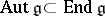# User:Maximilian Janisch/latexlist/Algebraic Groups/Trigonalizable element

triangular element

A trigonalizable element of the algebra $V$ of endomorphisms of a finite-dimensional vector space $V$ over a field $k$ is an element $X \in \text { End } V$ all eigenvalues of which belong to $k$. If $k$ is algebraically closed, then every element of $V$ is trigonalizable. For a trigonalizable element $x$ (and only for such an element) there exists a basis in $V$ with respect to which the matrix of the endomorphism $x$ is triangular (or, what is the same, there exists a complete flag in $V$ that is invariant with respect to $x$). A trigonalizable element has a Jordan decomposition over $k$. There exist a number of generalizations of the notion of a trigonalizable element in $V$ for the case that $V$ is infinite-dimensional (see ).

A trigonalizable element of a finite-dimensional algebra $4$ over a field $k$ is an element $x \in A$ such that the operator of right (or left, depending on the case under consideration) multiplication by $12$ is a trigonalizable element in the algebra $_ { k } A$. If $4$ is isomorphic to the algebra $V$ for some finite-dimensional vector space $V$ over $k$, then these two (formally distinct) definitions reduce to the same concept.

In Lie algebras, trigonalizability of an element $x \in A$ means trigonalizability of the endomorphism $a d _ { X }$ (where $\operatorname { ad } _ { x } ( y ) = [ x , y ]$). The set of all trigonalizable elements in a Lie algebra is, in general, not closed with respect to the operations of addition and commutation (for example, for $\mathfrak { g } [ ( 2 , R )$, the simple Lie algebra of real matrices of order 2 with trace 0). However, in the case of a solvable algebra $4$, this set is even a characteristic ideal of $4$.

A trigonalizable element in a connected finite-dimensional Lie group $k$ is an element $g \in G$ such that $Ad _ { g }$ is a trigonalizable element in $g$ (here $: G \rightarrow \text { Aut } g$ is the adjoint representation of the Lie group $k$ in the groupof automorphisms of its Lie algebra $8$). If $\operatorname { exp } : \mathfrak { g } \rightarrow G$ is the exponential mapping and $X \in q$ is a trigonalizable element (in the sense of 2)), then $p ( X )$ is a trigonalizable element of $k$. The converse is, in general, false.

Lie algebras and Lie groups all elements of which are trigonalizable are called trigonalizable algebras or groups, respectively, and also supersolvable Lie algebras, respectively (cf. Lie algebra, supersolvable; Lie group, supersolvable).

How to Cite This Entry:
Maximilian Janisch/latexlist/Algebraic Groups/Trigonalizable element. Encyclopedia of Mathematics. URL: http://encyclopediaofmath.org/index.php?title=Maximilian_Janisch/latexlist/Algebraic_Groups/Trigonalizable_element&oldid=44067